Courses

# Test Level 2: Solutions

## 30 Questions MCQ Test Chemistry Class 12 | Test Level 2: Solutions

Description
This mock test of Test Level 2: Solutions for Class 12 helps you for every Class 12 entrance exam. This contains 30 Multiple Choice Questions for Class 12 Test Level 2: Solutions (mcq) to study with solutions a complete question bank. The solved questions answers in this Test Level 2: Solutions quiz give you a good mix of easy questions and tough questions. Class 12 students definitely take this Test Level 2: Solutions exercise for a better result in the exam. You can find other Test Level 2: Solutions extra questions, long questions & short questions for Class 12 on EduRev as well by searching above.
QUESTION: 1

### Select correct statement -

Solution:

(A) NaCl → Na+ + Cl
ΔTb = Kb × ms
ΔTb = 2Kb × m
elavation of b.p. will be double in case of NaCl not b.p.
(B) Will be correct because b.p. elavation will be double here in comprasion to glucose.
(C) Elavation of b.p. is colligative property not b.p

QUESTION: 2

### Total vapour pressure of mixture of 1 mol volatile component A(pºA = 100 mmHg) and 3 mol of volatile component B (pºB = 60 mmHg) is 75 mm. For such case -

Solution:

As, we know that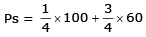= 25 + 45 mm Hg
= 70 mm Hg
To mm Hg < 75 mm Hg thus there is positive deviation from Raoults law,
so (A) is correct
(B) If v.p. is increasing boiling point will be lowered in that case
(C) is correct that force of attraction between A & B is smaller than that between A and A or between B and B.
So All statement are corrrect

QUESTION: 3

### At a given temperature, total vapour pressure in Torr of a mixture of volatile components A and B is given by P = 120 – 75 XB hence, vapour pressure of pure A and B respectively (in Torr) are -

Solution:

P = 120 – 75 × B
If XB = 1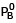= 120 – 70 = 45 mm Hg
If XB = 120 – 75 = 45 mm Hg
if  XB = 0    Ps =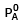= 120 mm Hg

QUESTION: 4

Van't Hoff factors of aqueous solutions of X, Y, Z are 1.8, 0.8 and 2.5. Hence, their (assume equal concentrations in all three cases) then correct order is :-

Solution:

As van't Holf factor increases RLVP increases i.e.,V,P. decreases y > x > z

QUESTION: 5

Decimolar solution of potassium ferricyanide, K3[Fe(CN)6] has osmotic pressure of 3.94 atm at 27ºC. Hence percent ionisation of the solute is -

Solution:

π = iCRT
K3[Fe(CN)6] = 3k+ + [Fe(CN)6]3–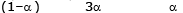i = (1 + 3α)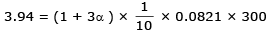α = 0.2
so  20%

QUESTION: 6

An aqueous solution of urea containing 18 g urea in 1500 cm3 of solution has a density of 1.052 g/cm3. If the molecular weight of urea is 60, then the molality of solution is-

Solution:

The correct answer is Option B.

Density is the ratio of mass to volume.
Density is 1.052 g/ml.
1500 ml of solution corresponds to
= 1.052 g/ml×1500ml
= 1578 g
Molar mass of urea is 60 g/mol. Number of moles of urea is the ratio of mass to molar mass. It is 18g / 60g/mol = 0.3mol

Molality of solution is the ratio of the number of moles of urea to the mass of solvent in kg.
Mass of water =1578 g − 18 g
=1560g
=1.560kg (as 1 kg = 1000g)
Molality = 0.3 / 1.560
= 0.192m

QUESTION: 7

2.56 g of sulphur in 100 g of CShas depression in f.p. of 0.010º, K= 0.1º (molal)-1. Hence, atomicity of sulphur is -

Solution: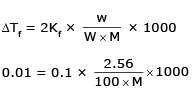M = 256
M = 8 × 32
Thus atomicity = 8

QUESTION: 8

Consider following solutions -

I : 1 M a glucose II. : 1 M a sodium chloride

III. : 1 M benzoic acid in benzene IV. : 1 M ammonium phosphate

Select incorrect statement -

Solution:

(A) All solutions are not isotonic because all solution are not at equal concentration so (A) is incorrect
(B) As benzoic acid dimerises so III is hypotonic of I, II, IV
(C) Is also correct as I, II & IV are hypertonic of III
(D) As ammoniun sulphate has maximum number of particale so it will be hypertonic of I, II, III

QUESTION: 9

The relationship between osmotic pressure at 273 K when 10 g glucose (P1) 10 g urea (P2) and 10 g sucrose (P3) are dissolved in 250 ml of water is -

Solution:

As number of moles is maximum in case urea > glucose > sucrose
π = CRT
It depends on number of moles so osmotic pressure
P2 > P1 > P3

QUESTION: 10

The osmotic pressure of a solution of benzoic acid dissolved in benzene is less than expected because-

Solution:

As benzoic acid dimerises so number of moles decreases so osmotic pressure of benzoic acid is less than benzene.

QUESTION: 11

Assuming each salt to be 90% dissociated, which of the following will have highest osmotic pressure

Solution:

Higher the number of particles higher will be osmoatic pressure so (A) will be answer in case of (D) precipitaiton will take place so number of particles will decrease.

QUESTION: 12

Which one of the following pairs of solution can we expect to be isotonic at the same temperature-

Solution:

Isotonic means equal osmotic pressure so, π1 = π2
i1C1RT = i2C2RT
so i1C1 = i2C2
In case of (D) both the volume of (i) & (C) are equal.

QUESTION: 13

For a solution containing non-volatile solute, the relative lowering of vapour pressure is 0.2. If the solution contains 5 moles in all, which of the following are true ?

I. Mole fraction of solute in the solution is 0.2
II. No. of moles of solute in the solution is 0.2
III. No. of moles of solvent in the solution is 4
IV. Mole fraction of solvent is 0.2 -

Solution: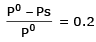As we know relative lowering of v.p. is equal to the mole fraction of the solute so (I) is correct it doesn’t depend upon the number of moles so (II) is wrong mole fraction of solvent will be 0.8 so number of moles of solvent will be 4 so (III) will be correct, (IV) will be also wrong so I & III will be correct

QUESTION: 14

A complex containing K+, Pt (IV) and Clis 100% ionised giving i = 3. Thus, complex is

Solution:

In option (B) oxidaton state of platinum is (iv)
x – 6 = –2
x = + 4

QUESTION: 15

If pK= -log K= 4, and K= Cαthen van't Hoff factor for weak monobasic acid when C = 0.01 M is-

Solution:

i = 1 + α
10–4 = Cα2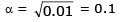i = 1 + 2 = 1 + 0.1 = 1.1

QUESTION: 16

pH of 1M HA (weak acid) is 2. Hence van't Hoff factor is -

Solution:

HA = H+ + A
(1 – α) α       α
PH = 2 = [H+]  : 10–2  = C2
a = 0.1
i = 1 + α = 1 + 0.1 = 1.01

QUESTION: 17

In which case van't Hoff factor is maximum

Solution:

(a) As we know
i = 1 + α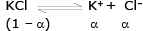i = 1 + α =  50 % dissociation
i = 1.5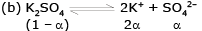1 + Zα ,  α = .4
i = 1.8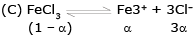1 + 3α
i = 1.9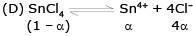1 + 4α
i = 1.8

QUESTION: 18

If 18 gram of glucose (C6H12O6) is present in 1000 gram of an aqueous solution of glucose it is said to be-

Solution:

18 gm of glucose means 0.1mole of glucose as it present in 1000 gm of solvent so it is 0.1 mole

QUESTION: 19

What is the molarity of H2SO4 solution that has a density of 1.84 gm/cc at 350C and contains 98% by weight-

Solution: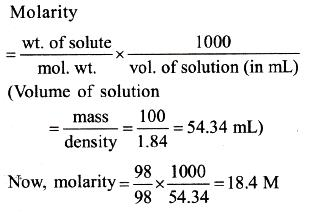QUESTION: 20

In order to prepare 100 cm3 of 0.250 M barium chloride solution the amount of BaCl2.2H2O required will be-

Solution:

Molality of BaCl2 = 0.1 × 0.25 = 0.025
by calculation we get the values of (D)

QUESTION: 21

25 mL of 3 M HCl were added to 75 mL of 0.05 M HCl. The molarity of HCl in the resulting solution is approximately-

Solution:

Mole of HCl = 25 × 3 +75 × 0.05 = 75 + 3.75 = 78.75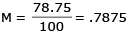QUESTION: 22

0.2 mole of HCl and 0.1 mole of CaCl2 were dissolved in water to have 500 ml of solution, the molarity of Cl¯ ions is-

Solution: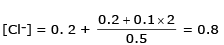QUESTION: 23

When 5.0 gram of BaCl2 is dissolved in water to have 106 gram of solution. The concentration of solution is-

Solution: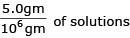so concentration of solution = 5 ppm

QUESTION: 24

For an ideal binary liquid solution with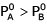, which relation between XA(molefraction of A in liquid phase) and YA (mole fraction of A in vapour phase) is correct?

Solution: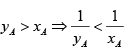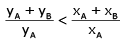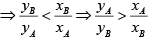QUESTION: 25

Mole fraction of A vapours above the solution in mixture of A and B(XA = 0.4) will be

[Given :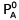= 100 mm Hg and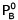= 200 mm Hg]

Solution: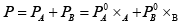= 40 + 120 = 160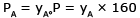π 40 = yA × 160
⇒ yA = ¼

QUESTION: 26

The exact mathematical expression of Raoult's law is

Solution:

The exact mathematical expression of Raoult's law is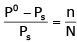Here, Po represents the vapour pressure of the pure solvent, P represents the vapour pressure of the solution, n represents the number of moles of solute and N represents the number of moles of the solvent.

QUESTION: 27

A mixture contains 1 mole of volatile liquid A ( = 100 mm Hg) and 3 moles of volatile liquid B(= 80 mmHg). If solution behaves ideally, the total vapour pressure of the distillate is

Solution: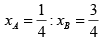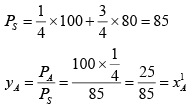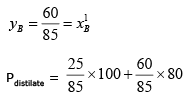= 85.88 mm Hg

QUESTION: 28

Which of the following aqueous solution will show maximum vapour pressure at 300 K?

Solution:

less no of particle of solute means maximum vapour pressure.

QUESTION: 29

The Van't Hoff factor for a dilute aqueous solution of glucose is

Solution:

Glucose does not dissociate
⇒ i = 1

QUESTION: 30

The correct relationship between the boiling points of very dilute solution of AlCl3(T1K) and CaCl2(T2K) having the same molar concentration is

Solution:

AlCl3 = no. of particle = 4
CaCl2 = no. of particle = 3
(vapour pressure of AlCl3) < (vapour pressure of CaCl2 solution)
TB.P. (AlCl3) > TB.P. (CaCl2)
T1 > T2